# 2.Prerequisites

`pip install numpypip install opencv-pythonpip install matplotlib`

# 5. Create or Download the Files

`import cv2from matplotlib import pyplot as pltimport mathimport numpy as npimport imutil`

# 6.Transformation Functions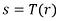Equation (1)

## 6.1.Logarithmic Transformation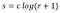Equation (2)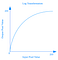Figure 1

## 6.2.Exponential Transformation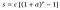Equation (3)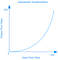Figure 2

## 6.3.Power-law(gamma) Transformation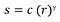Equation (4)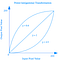Figure 3

# 7.Histogram Sliding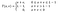Equation (5)

# 8.Contrast(Histogram) Stretching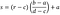Equation (6)

# 9.Histogram Equalization

## 9.1. The Classical Histogram Equalization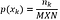Equation (7)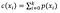Equation (8)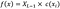Equation (9)
`1.Calculate probability for each intensity level and obtain pdf starting from 0 to L-1 using Eq. (7)2.Calculate cdf using Eq. (8)3.Change the intensity values of the input image with the new intensity values obtained using Eq. (9)4.Obtain output image`

## 9.2. Brightness Preserving Bi-histogram Equalization (BBHE)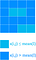Illustration of the BBHE method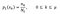Equation (10)Equation (11)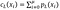Equation (12)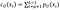Equation (13)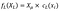Equation (14)Equation (15)
`1.Divide the image into lower and upper parts based on the mean2.Keep the locations of the pixels belong to lower and upper parts in hashmap(s)3.Calculate cdf and pdf for both of the two parts4.Apply the appropriate transformation function by looking at the values at hashmaps5.Obtain output image`

## 9.3. Equal Area Dualistic Sub-Image Histogram Equalization (DSIHE)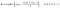Equation (16)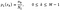Equation (17)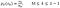Equation (18)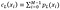Equation (19)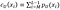Equation (20)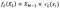Equation (21)Equation (22)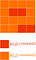Illustration of the DSIHE method
`1.Calculate the pdf and cdf of the input image using Eq. (7) and Eq.(8) respectively2.Using Eq. (16) find the median of the image and divide the image into lower and upper parts3.Keep the locations of the pixels belong to lower and upper parts in hashmap(s)3.Calculate cdf and pdf for both of the two parts4.Apply the appropriate transformation function by looking at the values at hashmaps5.Obtain output image`